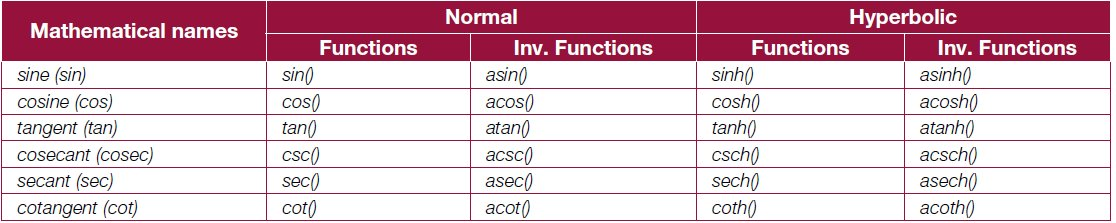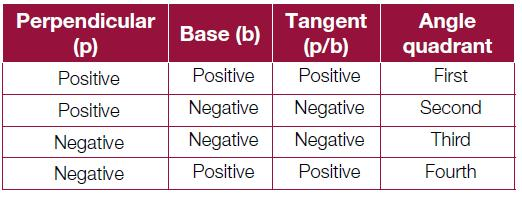# You Can Master Trigonometry with Maxima!

0
4535Maxima is a descendant of Macsyma, a breed of computer algebra systems, which was developed at MIT in the late 1960s. Owing to its open source nature, it has an active user community. This is the 17th article in the Mathematics in Open Source series, in which the author deals with fundamental trigonometric expressions.

Trigonometry first gets introduced to students of Standard IX through triangles. Thereafter, students have to wade through a jungle of formulae and tables. A good student is one who can instantly recall various trigonometric formulae. The idea here is not to be good at rote learning but rather to apply the formulae to get the various end results, assuming that you already know the formulae.

Fundamental trigonometric functions
Maxima provides all the familiar fundamental trigonometric functions, including the hyperbolic ones (see Table 1).Note that all arguments are in radians. And here follows a demonstration of a small subset of these:

```\$ maxima -q
(%i1) cos(0);
(%o1) 1
(%i2) cos(%pi/2);
(%o2) 0
(%i3) cot(0);
The number 0 isnt in the domain of cot
-- an error. To debug this try: debugmode(true);
(%i4) tan(%pi/4);
(%o4) 1
(%i5) string(asin(1));
(%o5) %pi/2
(%i6) csch(0);
The number 0 isnt in the domain of csch
-- an error. To debug this try: debugmode(true);
(%i7) csch(1);
(%o7) csch(1)
(%i8) asinh(0);
(%o8) 0
(%i9) string(%i * sin(%pi / 3)^2 + cos(5 * %pi / 6));
(%o9) 3*%i/4-sqrt(3)/2
(%i10) quit();```

Simplifications with special angles like %pi/ 10 and its multiples can be enabled by loading the ntrig package. Check the difference below before and after the package is loaded:

```\$ maxima -q
(%i1) string(sin(%pi/10));
(%o1) sin(%pi/10)
(%i2) string(cos(2*%pi/10));
(%o2) cos(%pi/5)
(%i3) string(tan(3*%pi/10));
(%o3) tan(3*%pi/10)
(%o4) /usr/share/maxima/5.24.0/share/trigonometry/ntrig.mac
(%i5) string(sin(%pi/10));
(%o5) (sqrt(5)-1)/4
(%i6) string(cos(2*%pi/10));
(%o6) (sqrt(5)+1)/4
(%i7) string(tan(3*%pi/10));
(%o7) sqrt(2)*(sqrt(5)+1)/((sqrt(5)-1)*sqrt(sqrt(5)+5))
(%i8) quit();```

A very common trigonometric problem is as follows: given a tangent value, find the corresponding angle. A common challenge is that for every value, the angle could lie in two quadrants. For a positive tangent, the angle could be in the first or the third quadrant, and for a negative value, the angle could be in the second or the fourth quadrant. So, atan() cannot always calculate the correct quadrant of the angle. How then, can we know what it is, exactly? Obviously, we need some extra information, say, the actual values of the perpendicular (p) and the base (b) of the tangent, rather than just the tangent value. With that, the angle location could be tabulated as follows:This functionality is captured in the atan2() function, which takes two arguments, p and b, and thus does provide the angle in the correct quadrant, as per the table above. Along with this, the infinities of tangent are also taken care of. Heres a demo:

```\$ maxima -q
(%i1) atan2(0, 1); /* Zero */
(%o1) 0
(%i2) atan2(0, -1); /* Zero */
(%o2) %pi
(%i3) string(atan2(1, -1)); /* -1 */
(%o3) 3*%pi/4
(%i4) string(atan2(-1, -1)); /* 1 */
(%o4) -3*%pi/4
(%i5) string(atan2(-1, 0)); /* - Infinity */
(%o5) -%pi/2
(%i6) string(atan2(5, 0)); /* + Infinity */
(%o6) %pi/2
(%i7) quit();```

Trigonometric identities
Maxima supports many built-in trigonometric identities and you can add your own as well. The first one that we will look at is the set dealing with integral multiples and factors of %pi. Lets declare a few integers and then play around with them:

```\$ maxima -q
(%i1) declare(m, integer, n, integer);
(%o1) done
(%i2) properties(m);
(%o2) [database info, kind(m, integer)]
(%i3) sin(m * %pi);
(%o3) 0
(%i4) string(cos(n * %pi));
(%o4) (-1)^n
(%i5) string(cos(m * %pi / 2)); /* No simplification */
(%o5) cos(%pi*m/2)
(%i6) declare(m, even); /* Will lead to simplification */
(%o6) done
(%i7) declare(n, odd);
(%o7) done
(%i8) cos(m * %pi);
(%o8) 1
(%i9) cos(n * %pi);
(%o9) - 1
(%i10) string(cos(m * %pi / 2));
(%o10) (-1)^(m/2)
(%i11) string(cos(n * %pi / 2));
(%o11) cos(%pi*n/2)
(%i12) quit();```

Next is the relation between the normal and the hyperbolic trigonometric functions:

```\$ maxima -q
(%i1) sin(%i * x);
(%o1) %i sinh(x)
(%i2) cos(%i * x);
(%o2) cosh(x)
(%i3) tan(%i * x);
(%o3) %i tanh(x)
(%i4) quit();```

By enabling the option variable halfangles, many half-angle identities come into play. To be specific, sin(x/2) gets further simplified in the (0, 2 * %pi) range, and cos(x/2) gets further simplified in the (-%pi/2, %pi/2) range. Check out the differences, before and after enabling the option variable, along with the range modifications, in the examples below:

```\$ maxima -q
(%i1) string(2*cos(x/2)^2 - 1); /* No effect */
(%o1) 2*cos(x/2)^2-1
(%i2) string(cos(x/2)); /* No effect */
(%o2) cos(x/2)
(%i3) halfangles:true; /* Enabling half angles */
(%o3) true
(%i4) string(2*cos(x/2)^2 - 1); /* Simplified */
(%o4) cos(x)
(%i5) string(cos(x/2)); /* Complex expansion for all x */
(%o5) (-1)^floor((x+%pi)/(2*%pi))*sqrt(cos(x)+1)/sqrt(2)
(%i6) assume(-%pi < x, x < %pi); /* Limiting x values */ (%o6) [x > - %pi, x < %pi]
(%i7) string(cos(x/2)); /* Further simplified */
(%o7) sqrt(cos(x)+1)/sqrt(2)
(%i8) quit();```

Trigonometric expansions and simplifications
Trigonometry is full of multiples of angles, the sums of angles, the products and the powers of trigonometric functions, and the long list of relations between them. Multiples and sums of angles fall into one category. The products and powers of trigonometric functions fall in another category. Its very useful to do conversions from one of these categories to the other one, to crack a range of simple and complex problems catering to a range of requirementsfrom basic hobby science to quantum mechanics. trigexpand() does the conversion from multiples and sums of angles to products and powers of trigonometric functions. trigreduce() does exactly the opposite. Heres a small demo:

```\$ maxima -q
(%i1) trigexpand(sin(2*x));
(%o1) 2 cos(x) sin(x)
(%i2) trigexpand(sin(x+y)-sin(x-y));
(%o2) 2 cos(x) sin(y)
(%i3) trigexpand(cos(2*x+y)-cos(2*x-y));
(%o3) - 2 sin(2 x) sin(y)
(%i4) trigexpand(%o3);
(%o4) - 4 cos(x) sin(x) sin(y)
(%i5) string(trigreduce(%o4));
(%o5) -2*(cos(y-2*x)/2-cos(y+2*x)/2)
(%i6) string(trigsimp(%o5));
(%o6) cos(y+2*x)-cos(y-2*x)
(%i7) string(trigexpand(cos(2*x)));
(%o7) cos(x)^2-sin(x)^2
(%i8) string(trigexpand(cos(2*x) + 2*sin(x)^2));
(%o8) sin(x)^2+cos(x)^2
(%i9) trigsimp(trigexpand(cos(2*x) + 2*sin(x)^2));
(%o9) 1
(%i10) quit();```

In %o5 above, you might have noted that the 2s could have been cancelled for further simplification. But that is not the job of trigreduce(). For that we have to apply the trigsimp() function as shown in %i6. In fact, many other trigonometric identities-based simplifications are achieved using trigsimp(). Check out the %i7 to %o9 sequences for another such example.